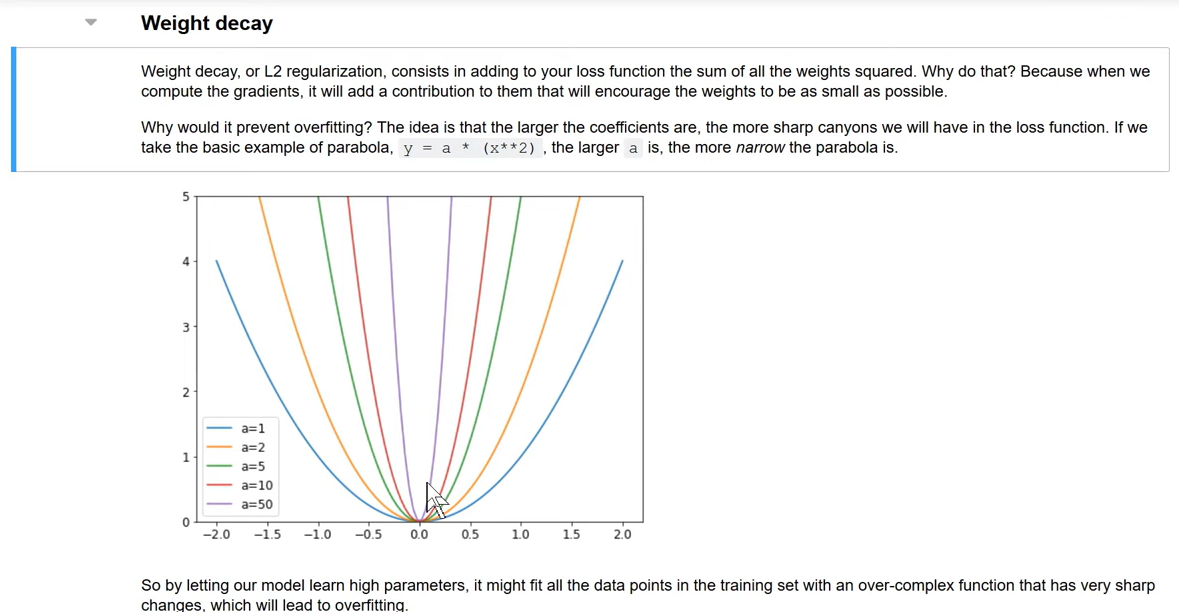Question 1/10 fast.ai v4 lecture 7

# How does L2 regularization (weight decay) work?

Answer

Regularization reduces the capacity of a model. This makes it less likely that a model will overfit.

The capacity of a model is how much space it has to find answers - if it can find any answer anywhere, those answers can include memorizing the dataset. One way to handle this would be to decrease the number of parameters or simplify the architecture in some other way, but generally speaking (especially in the deep learning context) this ends up biasing the model towards very simple shapes.

There is a better way to do this. Rather than arbitrarily reducing the number of parameters, we instead try to force the parameters to be smaller unless they are really required to be big.

We achieve this by adding the sum of all the parameters squared to our loss.Relevant part of lecture